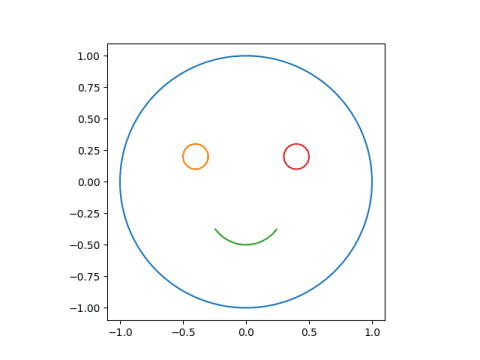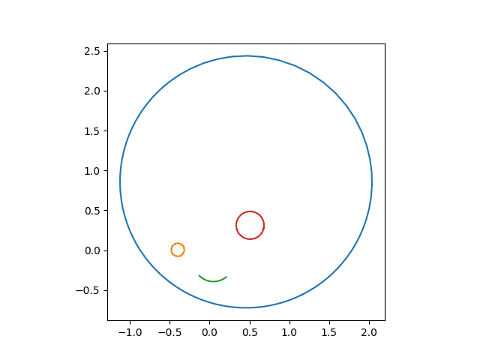# Constructing bilinear transformations

The previous post was a visual introduction to bilinear transformations, a.k.a. Möbius transformations or fractional linear transformations. This post is a short follow-up focused more on calculation.

A bilinear transformation f has the form## Inverse

The inverse of f is given byThe transformation f is defined everywhere except at z = –d/c, and its inverse is defined everywhere except at w = a/c.

So f takes the complex plane minus one point to the complex plane minus one point. Or an elegant way of thinking about it is to think of f and g as functions on a sphere by adding a point at infinity. Then we say## Determining by three points

Bilinear transformations have three degrees of freedom. That is, you can pick three values in the domain and specify three places for them to go in the range. The unique bilinear transform sending z1, z2, and z3 to w1, w2, and w3 is given byPlug in your constants and solve for w = f(z).

Update: Here are explicit formulas for the coefficients.

## Example

For example, let’s look at the smiley face example from the previous post.We’ll pick three points on the face and three places for them to go.

Let’s say we want the center of the face to stay put, mapping 0 to 0. Next let’s pick two places for the center of the eyes to go. These are at
±0.4+.2ji. Say we want the left eye to go down a little to -0.4 and the right eye to go up and over a little to 0.5 + 0.3i.

I used Mathematica to solve for the parameters.

    {z1, z2, z3} = {0, -2/5 + I/5, 2/5 + I/5}
{w1, w2, w3} = {0, -2/5, 1/2 + 3 I/10}
Solve[(w - w2) (w3 - w1)/((w - w1) (w3 - w2)) ==
(z - z2) (z3 - z1)/((z - z1) (z3 - z2)), w]


This says the parameters are, in Python notation,

    a = -72 - 16j
b = 0
c = 30 - 35j
d = -75


Using the code from the previous post we can verify that this transformation does what we designed it to do.

    print(mobius(0, a, b, c, d))
print(mobius(-0.4 + 0.2j, a, b, c, d))
print(mobius(0.4 + 0.2j, a, b, c, d))


and we can take a look at the result.